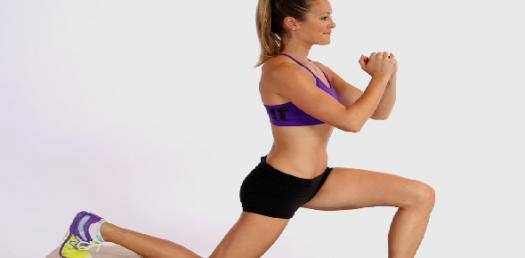# Comp2mm STAT Warm Up

36 Questions | Attempts: 82
ShareSettings• 1.
Methods of organizing, summarizing, and presenting data in an informative way
• A.

Descriptive Statistics

• B.

Inferential Statistics

• C.

Informative Statistics

• D.

Theoretical Statistics

• E.

Statistics

• 2.
The methods used to determine something about a population, based on a sample
• A.

Descriptive Statistics

• B.

Inferential Statistics

• C.

Informative Statistics

• D.

Theoretical Statistics

• E.

Sampling

• 3.
The entire set of individuals or objects of interest or the measurements obtained from all individuals or objects of interest
• A.

Statistics

• B.

Observation

• C.

Sample

• D.

Population

• E.

Portion

• 4.
A portion or part of the population of interest
• A.

Statistics

• B.

Observation

• C.

Sample

• D.

Population

• E.

Portion

• 5.
According to the latest Pulse Asia which surveyed 2,400 Filipinos, 95% of the Filipinos do not want Boy Abunda to be the secretary of the Department of Tourism. This is an example of ____________ statistics.Ma
• 6.
Marital status, gender, and brand names are examples ______________ variables.
• 7.
Quantitative variables which are ___________ can assume only certain values, and there are usually gaps between the values.
• 8.
It can assume any values within a specific range
• A.

Quantitative variables

• B.

Discrete variables

• C.

Flowing variables

• D.

Continuous variables

• E.

Qualitative variables

• 9.
_________level data can only be classified. E.g. Cellphone networks
• A.

Nominal

• B.

Ordinal

• C.

Interval

• D.

Ratio

• E.

Proportion

• 10.
___________ level data can be ranked. E.g. ITEO Faculty Evaluation
• A.

Nominal

• B.

Ordinal

• C.

Interval

• D.

Ratio

• E.

Proportion

• 11.
________ level data includes the characteristics of the ordinal level, but in addition, the difference between values is a constant size. E.g. Income bracket
• A.

Nominal

• B.

Ordinal

• C.

Interval

• D.

Ratio

• E.

Proportion

• 12.
A grouping of data into mutually exclusive classes showing the number of observations in each is called ________________.
• 13.
The range between classes/group is called _________
• A.

Raw data

• B.

Interval

• C.

Distribution

• D.

Frequency

• E.

Count

• 14.
The highest and lowest value of a class or group is called
• A.

Class constraint

• B.

Class limit

• C.

Class range

• D.

Class width

• E.

Class interval

• 15.
_____________ shows the fraction of the total number of observations in each class.
• A.

Percentage

• B.

Ratio

• C.

Proportion

• D.

Relative frequency

• E.

Frequency distribution

• 16.
One advantage of using stem-and-leaf technique is
• A.

It maintains the identity of the observation

• B.

It is easier to execute

• C.

It is more accurate

• D.

It is widely used and accepted

• E.

It looks good in presentations

• 17.
A characteristic of the population
• A.

Inference

• B.

Parameter

• C.

Statistic

• D.

Mean

• E.

Average

• 18.
A character of the sample
• A.

Inference

• B.

Parameter

• C.

Statistic

• D.

Mean

• E.

Average

• 19.
The average of the sample
• A.

Sample mean

• B.

Population mean

• C.

Sample median

• D.

Population mode

• E.

Mean

• 20.
The midpoint values after they have been in ordered from the smallest to largest, or largest to smallest.
• A.

Mean

• B.

Median

• C.

Mode

• D.

Average

• E.

Middle observation

• 21.
The value of the observation that appears most frequently
• A.

Mean

• B.

Median

• C.

Mode

• D.

Average

• E.

Middle observation

• 22.
The bell-shaped curve is called ________ curve.
• 23.
If the curve is positively skewed, it means the the curve is skewed to the right.
• A.

True

• B.

False

• C.

Neither true nor false

• 24.
The arithmetic mean of the squared deviations from the mean.
• A.

Variance

• B.

Dispersion

• C.

Standard Error

• D.

Range

• E.

• 25.
The square root of the variance
• A.

Square-variance

• B.

Dispersion

• C.

Standard Error

• D.

Range

• E.Back to top